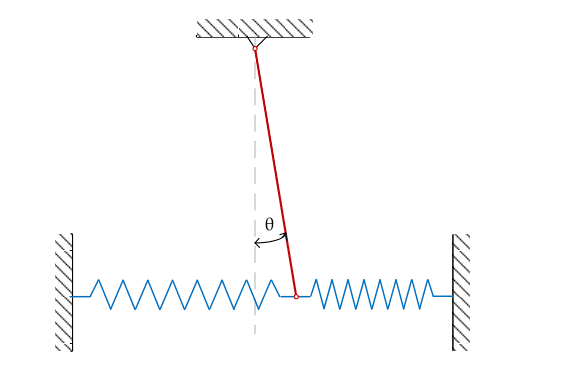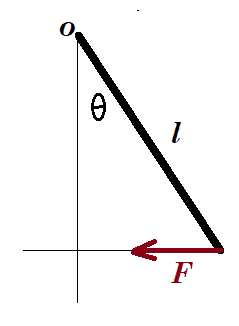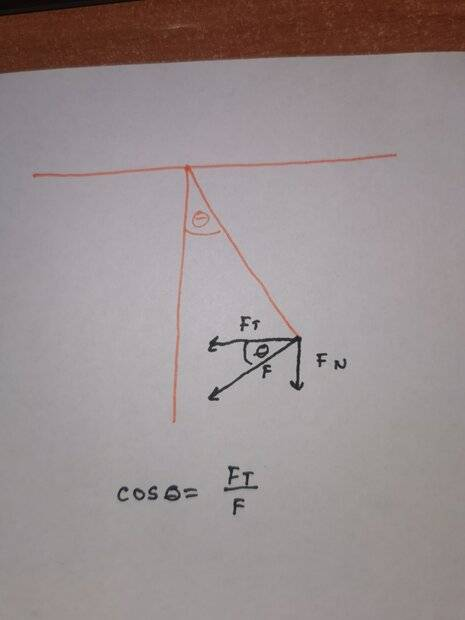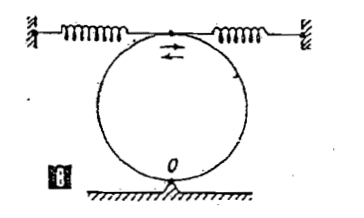# Torque calculation for a compound pendulum

charon_9
Homework Statement:
Newton's second law on a compound pendulum
Relevant Equations:
I*α = ΣM, F=-kx, G=mgI need to write an equation for Newton's second law for the above system, where k1=k2 (both springs are the same). The red line represents a bar with m=2kg, l=2m.

I know that I*α = M1 + M2 + M3

If we displace the bar by x, we get the angle of displacement theta.

M1=M2=-k*x

I know that, judging by the picture, tan(Θ)=x/l, thus x=tan(Θ)*l.
However, my workbook states that M1=-k*tan(Θ)*l*l*cos(Θ). Where did the l*cos(Θ) come from?

Homework Helper
Gold Member
Setting the workbook aside for the moment, how would you write Newton's second law for this arrangement?

Homework Helper
Gold Member
2022 Award
Where did the l*cos(Θ) come from?
Strictly speaking, the springs will not remain horizontal. As the rod swings, its end will rise slightly. So is ##x=l\tan(\theta), l\sin(\theta), ## or ##2l\sin(\theta/2)##?
I'm sure we will be doing a small angle approximation, so it is not going to matter. I.e. ##\cos(\theta)\approx 1##.

•berkeman
charon_9
Strictly speaking, the springs will not remain horizontal. As the rod swings, its end will rise slightly. So is ##x=l\tan(\theta), l\sin(\theta), ## or ##2l\sin(\theta/2)##?
I'm sure we will be doing a small angle approximation, so it is not going to matter. I.e. ##\cos(\theta)\approx 1##.
Yes, we're always using an approximation that $$cos(\theta) = 1$$ for small angles.
Thing is, I can understand that $$M_1 = kx \cdot r$$, where I already figured out that $$x=l\tan{\theta}$$

However, I really can't figure out where they got the $$l\cos{\theta}$$ in

$$M_1=-kl\tan{\theta} \cdot l\cos{\theta}$$

charon_9
Setting the workbook aside for the moment, how would you write Newton's second law for this arrangement?

Well,

$$I\alpha = M_1 + M_2 + M_3$$ where $$M_1 = M_2$$.

$$M_1 = M_2 = -k \cdot l\tan{\theta}$$

Now I get stuck figuring out the radius part. I have no clue where they got the cosine from.

Homework Helper
Gold Member
However, I really can't figure out where they got the $$l\cos{\theta}$$
For the figure shown below, how would you express the moment (torque) about O of the force F in terms of F, l, and ##\theta##?(They appear to be ignoring the tilt of the springs pointed out by @haruspex .)

Homework Helper
Gold Member
Now I get stuck figuring out the radius part. I have no clue where they got the cosine from.
To add to what @TSny posted, the picture that you posted in #1 is misleading. Strictly speaking, as the rod swings to some angle, the springs will no longer be horizontal. However, in the small angle approximation, you have ##l\cos\!\theta \approx l## and it wouldn't matter that the springs are not horizontal.

charon_9
For the figure shown below, how would you express the moment (torque) about O of the force F in terms of F, l, and ##\theta##?
View attachment 321364

(They appear to be ignoring the tilt of the springs pointed out by @haruspex .)
Thank you for taking the time to answer.

Wasn't it $$M=r \times F = r \cdot F \sin{\theta}$$

Homework Helper
Gold Member
Wasn't it $$M=r \times F = r \cdot F \sin{\theta}$$
##|\vec r \times \vec F| = rF \sin\phi##, where ##\phi## is the angle between ##\vec r## and ##\vec F##. Is the angle ##\theta## is the diagram the same as the angle between ##\vec r## and ##\vec F##?

Last edited:
charon_9
To add to what @TSny posted, the picture that you posted in #1 is misleading. Strictly speaking, as the rod swings to some angle, the springs will no longer be horizontal. However, in the small angle approximation, you have ##l\cos\!\theta \approx l## and it wouldn't matter that the springs are not horizontal.
Sorry for posting a misleading picture, that's what I have been provided with by my school. I'm once again sorry.
I did some testing so please tell me if I'm right or wrong.
Because the springs are a bit elevated. their force F has a vertical component and a component in the direction of $$\theta$$. We need the component in the direction of theta, so $$G_t = G\cos{\theta}$$.
As for the ##l##, it is the radius, or the distance from the tip of the bar to the point about which it oscillates. Is this correct?

Homework Helper
Gold Member
2022 Award
Sorry for posting a misleading picture
No, that's ok, we know it is not your picture. I think @kuruman was just making sure you were not being misled by it.

Because the springs are a bit elevated. their force F has a vertical component and a component in the direction of $$\theta$$. We need the component in the direction of theta, so $$G_t = G\cos{\theta}$$.
G? Gt? What has that to do with the tension in the springs?
The elevation of the springs will not be to angle ##\theta##. It will depend on the spring length, so taking them to be extremely long gets rid of that problem.
As for the l, it is the radius, or the distance from the tip of the bar to the point about which it oscillates. Is this correct?
Yes.

Homework Helper
Gold Member
No, that's ok, we know it is not your picture. I think @kuruman was just making sure you were not being misled by it.
Yes.

charon_9
No, that's ok, we know it is not your picture. I think @kuruman was just making sure you were not being misled by it.

G? Gt? What has that to do with the tension in the springs?
The elevation of the springs will not be to angle ##\theta##. It will depend on the spring length, so taking them to be extremely long gets rid of that problem.

Yes.
Sorry. I was quick to reply so I mistook ##G## for ##F##, the elastic force.

This is my guess. Let's say the springs tilt up for a small angle. This means that the elastic force has a vertical component to it (in the negative direction of the y axis in an XY plane). We need the other component - the one that "pulls" to the left.I didn't draw the springs in order to not make the sketch a mess. I just named the components as such, where ##t## would be tangential and ##n## would be perpendicular.

Homework Helper
Gold Member
2022 Award
Let's say the springs tilt up for a small angle. This means that the elastic force has a vertical component to it (in the negative direction of the y axis in an XY plane). We need the other component - the one that "pulls" to the left.

View attachment 321374
As I posted, the angle the springs make to the horizontal will not be the same angle the rod makes to the vertical. To see that, try including the springs in the diagram, making them much longer than the rod.
Because we don’t know the lengths of the springs, we cannot know the angle they make.

charon_9
As I posted, the angle the springs make to the horizontal will not be the same angle the rod makes to the vertical. To see that, try including the springs in the diagram, making them much longer than the rod.
Because we don’t know the lengths of the springs, we cannot know the angle they make.
Okay, thanks. I have one more question, though. I decided not to open a new thread, but please tell me if I need to.

I took this photo from a very old textbook, where it asked us to write the same equation for this system, where the mass of the disc is ##M=2##, and ##k=37.5## for both springs. The solution says that
$$I\alpha = kx \cdot 2R$$

$$x = 2R \cdot \tan{\theta}$$

$$I \alpha = -4kR^2\tan{\theta}$$

I now have two questions:

1. Why did they only include the torque for one spring? Shouldn't it be ##2 \cdot -4kR^2\tan{\theta}##, like in the first example?

2. Why isn't the torque for the gravitational force included, also like in the first example?Homework Helper
Gold Member
2022 Award
Okay, thanks. I have one more question, though. I decided not to open a new thread, but please tell me if I need to.

I took this photo from a very old textbook, where it asked us to write the same equation for this system, where the mass of the disc is ##M=2##, and ##k=37.5## for both springs. The solution says that
$$I\alpha = kx \cdot 2R$$

$$x = 2R \cdot \tan{\theta}$$

$$I \alpha = -4kR^2\tan{\theta}$$

I now have two questions:

1. Why did they only include the torque for one spring? Shouldn't it be ##2 \cdot -4kR^2\tan{\theta}##, like in the first example?

2. Why isn't the torque for the gravitational force included, also like in the first example?
View attachment 321377
No idea. Both look like mistakes to me. Leaving out gravity would have made sense if the disc were rolling back and forth on a flat surface, but it is clearly mounted on a pivot.